# Octave – Basics of Plotting Data

Octave has some in-built functions for visualizing the data. Few simple plots can give us a better way to understand our data. whenever we perform a learning algorithm on an Octave environment, we can get a better sense of that algorithm and analyze it. Octave has lots of simple tools that we can use for a better understanding of our algorithm.
In this tutorial, we are going to learn how to plot data for better visualization and understanding it in the Octave environment.

Example 1 : Plotting a sine wave using the `plot()` and and `sin()` function:

 `% var_x for the y-axis ` `var_x = [0:0.01:1]; ` ` `  `% var_y for the y-axis ` `var_y = sin(4 * pi * var); ` ` `  `% plotting the graph ` `plot(var_x, var_y); `

Output :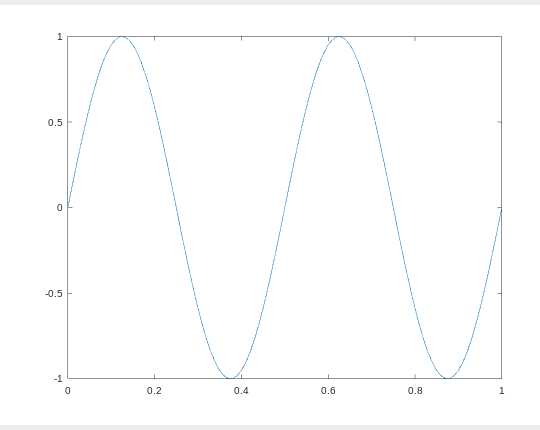Example 2 : Plotting a cosine wave using the `plot()` and and `cos()` function:

 `% var_x for the y-axis ` `var_x = [0:0.01:1]; ` ` `  `% var_y for the y-axis ` `var_y = cos(3 * pi * var); ` ` `  `% plotting the graph ` `plot(var_x, var_y); `

Output :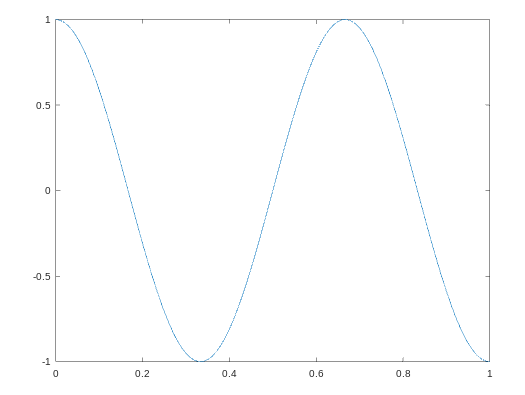Example 3 : We can plot, one plot over another plot by holding the previous plot with the `hold on` command.

 `% declaring variable var_x ` `var_x = [0:0.01:1]; ` ` `  `% declaring variable var_y1 ` `var_y1 = sin(4 * pi * var); ` ` `  `% declaring variable var_y2 ` `var_y2 = cos(3 * pi * var); ` ` `  `% plot var_x with var_y1 ` `plot(var_x, var_y1); ` ` `  `% hold the above plot or figure ` `hold on; ` ` `  `% plot var with var_y2 with red color ` `plot(var_x, var_y2, ``'r'``); `

Output :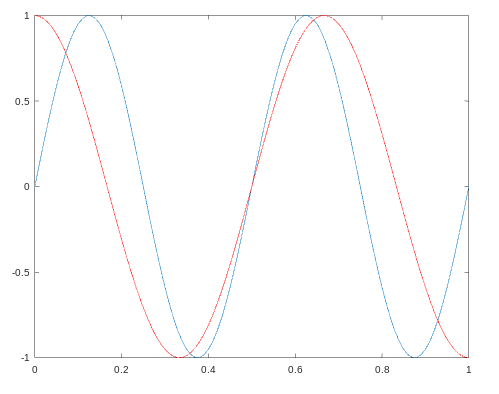Example 4 : We can add labels for the x-axis and the y-axis along with the `legends` and `title` with the below code.

 `% declaring variable var_x ` `var_x = [0:0.01:1]; ` ` `  `% declaring variable var_y1 ` `var_y1 = sin(4 * pi * var); ` ` `  ` `  `% declaring variable var_y2 ` `var_y2 = cos(3 * pi * var); ` ` `  `% plot var_x with var_y1 ` `plot(var_x, var_y1); ` ` `  `% hold the above plot or figure ` `hold on; ` ` `  `% plot var with var_y2 with red color ` `plot(var_x, var_y2, ``'r'``); ` ` `  `% adding label to the x-axis ` `xlabel(``'time'``); ` ` `  `% adding lable to the y-axis ` `ylabel(``'value'``); ` ` `  `% adding title for the plot ` `title(``'my first plot'``); ` ` `  `% add legends for these 2 curves ` `legend(``'sin'``, ``'cos'``); `

Output :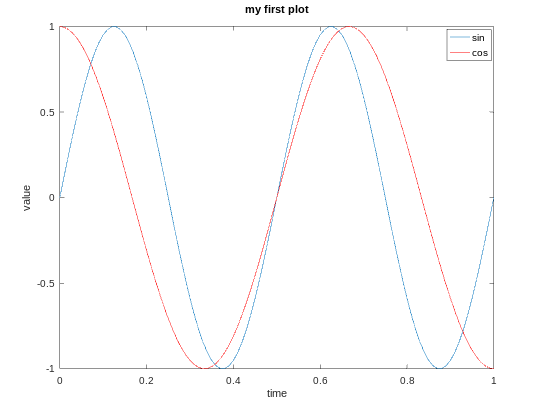Example 5 : We can also plot data on different figures.

 `% declaring variable var_x ` `var_x = [0:0.01:1]; ` `  `  `% declaring variable var_y1 ` `var_y1 = sin(4 * pi * var); ` `  `  `% declaring variable var_y2 ` `var_y2 = cos(3 * pi * var); ` ` `  `% plot var_x and var_y1 on figure 1 ` `figure(1);  ` `plot(var_x,var_y); ` ` `  `% plot var_x and var_y2 on figure 2 ` `figure(2);  ` `plot(var_x,var_y2); `

Output :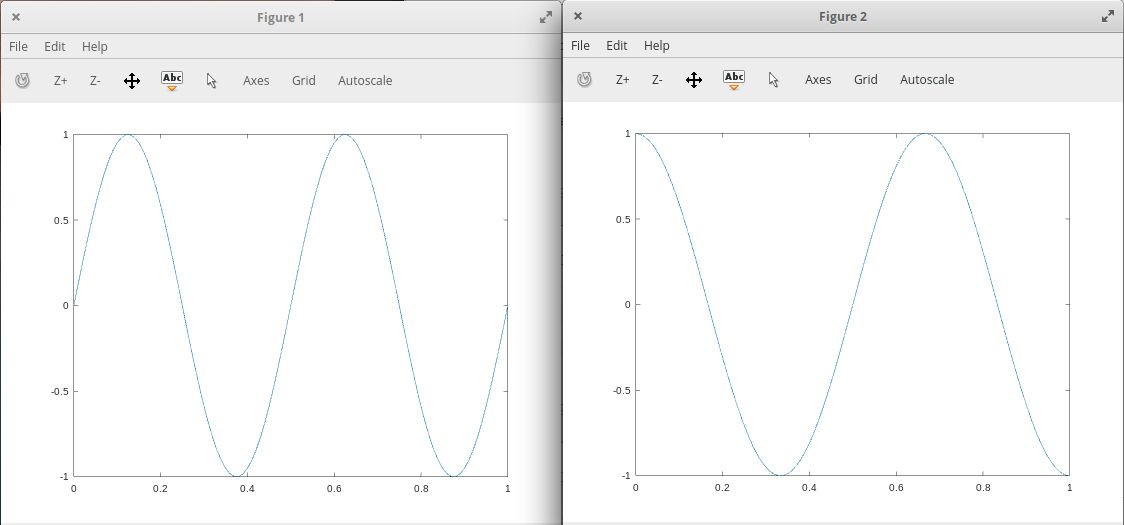Example 6 : We can divide a figure into a m x n grid using the `subplot()` function. In the below code the first 2 parameter shows m and n and 3rd parameter is the grid count from top to left.

 `% var_x for the y-axis ` `var_x = [0:0.01:1]; ` `  `  `% var_y for the y-axis ` `var_y = sin(4 * pi * var); ` ` `  `% plot the var_x and var_y on a 3x3 grid  ` `% at 4 position counting from top to left ` `subplot(3, 3, 4), plot(var_x, var_y); `

Output :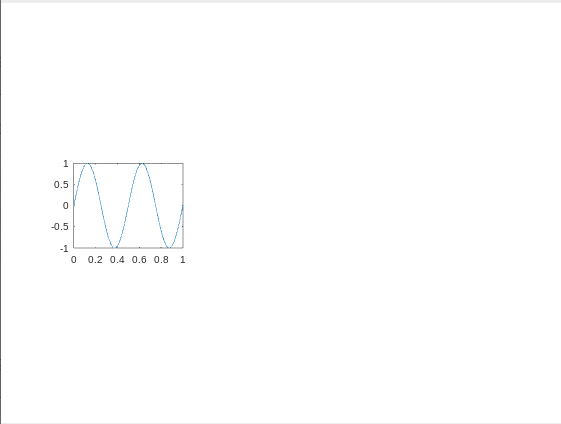Example 7 : We can change the `axis` values of any plot using the `axis()` function.

 `% declaring variable var_x ` `var_x = [0:0.01:1]; ` `  `  `% declaring variable var_y1 ` `var_y1 = sin(4 * pi * var); ` `  `  `% declaring variable var_y2 ` `var_y2 = cos(3 * pi * var); ` `  `  `% plot var_x with var_y1 ` `plot(var_x, var_y1); ` `  `  `% hold the above plot or figure ` `hold on; ` `  `  `% plot var with var_y2 with red color ` `plot(var_x, var_y2, ``'r'``); ` ` `  `% adding label to the x-axis ` `xlabel(``'time'``); ` ` `  `% adding lable to the y-axis ` `ylabel(``'value'``); ` `  `  `% adding title for the plot ` `title(``'my first plot'``); ` `  `  `% add legends for these 2 curves ` `legend(``'sin'``, ``'cos'``); ` ` `  `% first 2 parameter sets the x-axis  ` `% and next 2 will set the y-axis ` `axis([0.5 1 -1 1]) `

Here the first 2 parameters shows the range of the x-axis and the next 2 parameters shows the range of the y-axis.
Output :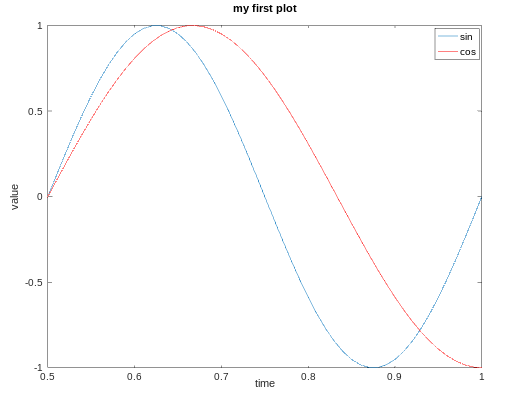Example 8 : We can save our plots in our present working directory :

 `print -dpng ``'plot.png'`

In order to print this plot at our desired location, we can use cd with it as shown below :

 `cd ``'/home/dikshant/Documents'``; print -dpng ``'plot.png'`

We can close a figure/plot using the `close `command.

Example 9 : We can visualize a matrix using the `imagesc(`) function.

 `% creating a 10x10 magic matrix ` `matrix = magic(10) ` ` `  `% plot the matrix ` `imagesc(matrix) `

Output :

```matrix =

92    99     1     8    15    67    74    51    58    40
98    80     7    14    16    73    55    57    64    41
4    81    88    20    22    54    56    63    70    47
85    87    19    21     3    60    62    69    71    28
86    93    25     2     9    61    68    75    52    34
17    24    76    83    90    42    49    26    33    65
23     5    82    89    91    48    30    32    39    66
79     6    13    95    97    29    31    38    45    72
10    12    94    96    78    35    37    44    46    53
11    18   100    77    84    36    43    50    27    59
```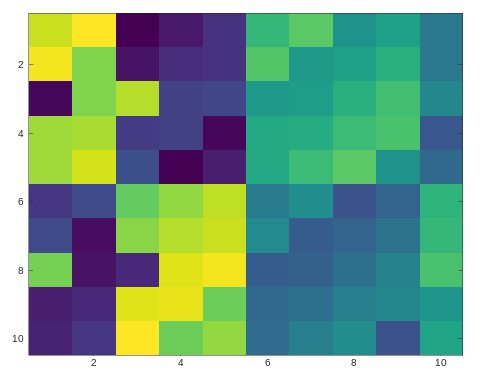The above plot is of 10×10 grid, each grid represents a value with a color. The same color value results in the same color.

We can also make a color bar with this plot to see which value corresponds to which color using the `colorbar` command. We can use multiple commands at a time by separating them with a comma(,) in Octave environment.

 `% creating a 10x10 magic matrix ` `matrix = magic(10) ` ` `  `% plot this matrix with showing colorbar on the right of it ` `imagesc(matrix), colorbar; `

Output :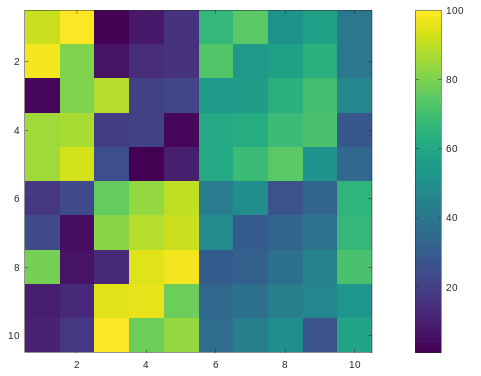Drawing the magic square with a gray-scale colormap :

 `% creating a 10x10 magic matrix ` `matrix = magic(10) ` ` `  `% plot this matrix with colorbar and gray colormap ` `imagesc(matrix), colorbar, colormap gray; `

Output :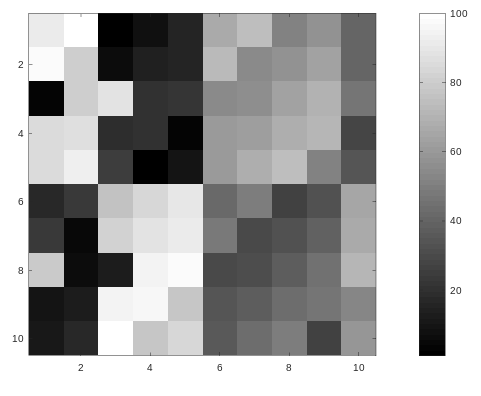My Personal Notes arrow_drop_upCheck out this Author's contributed articles.

If you like GeeksforGeeks and would like to contribute, you can also write an article using contribute.geeksforgeeks.org or mail your article to contribute@geeksforgeeks.org. See your article appearing on the GeeksforGeeks main page and help other Geeks.

Please Improve this article if you find anything incorrect by clicking on the "Improve Article" button below.

Article Tags :

Be the First to upvote.

Please write to us at contribute@geeksforgeeks.org to report any issue with the above content.